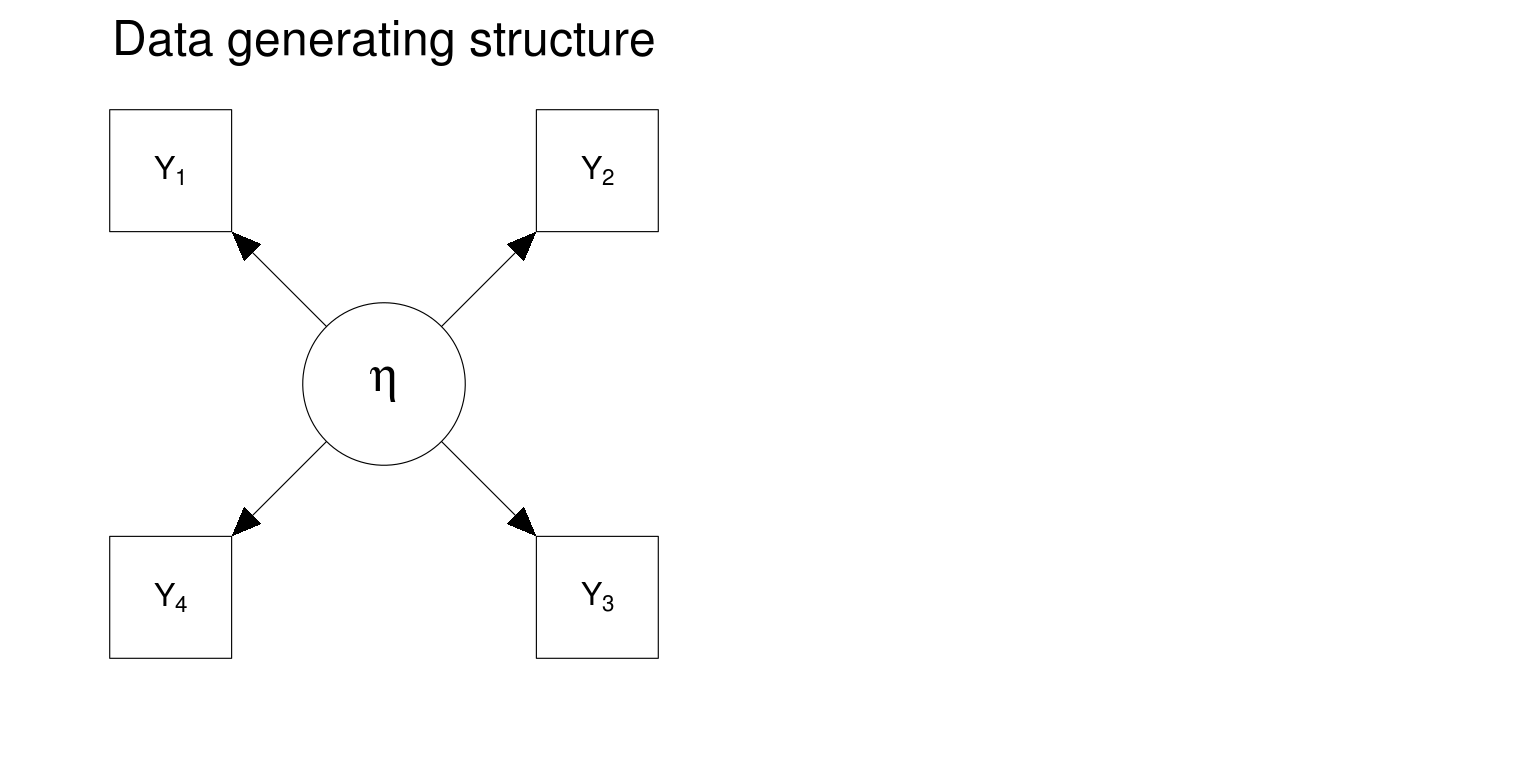## Local independence

• Are "Get angry easily" and "Get irritated easily" locally independent given Neuroticism?
• Are "Don't talk a lot" and "Find it difficult to approach others" locally independent given Extroversion?
• Are "Am indifferent to the feelings of others" and "Inquire about others' well-being" locally independent given Agreeableness?
• Are "Avoid difficult reading material" and "Will not probe deeply into a subject" locally independent given Openness to Experience?
• Are "Do things in a half-way manner" and "Waste my time" locally independent given Conscientiousness?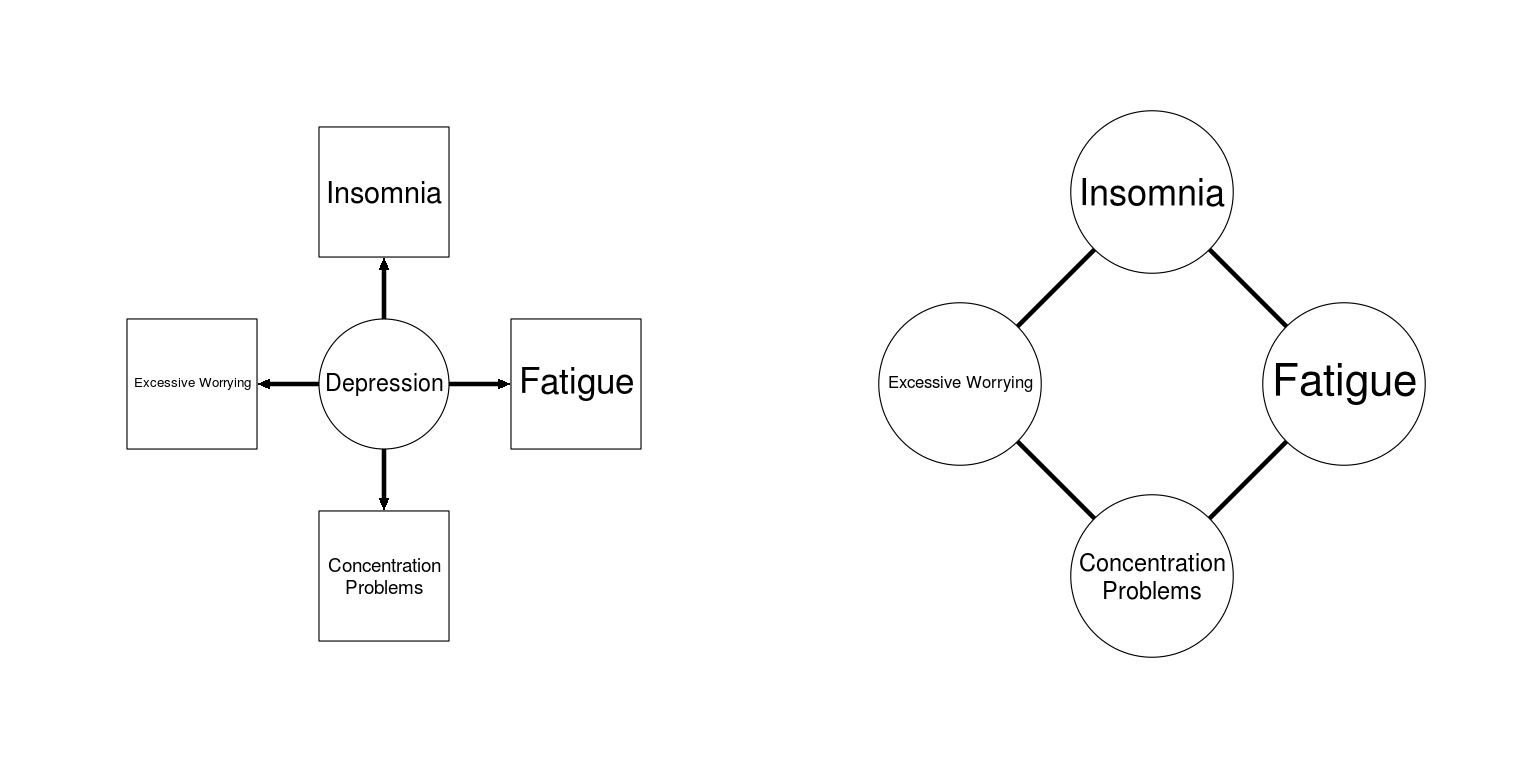## Network Psychometrics Ecosystem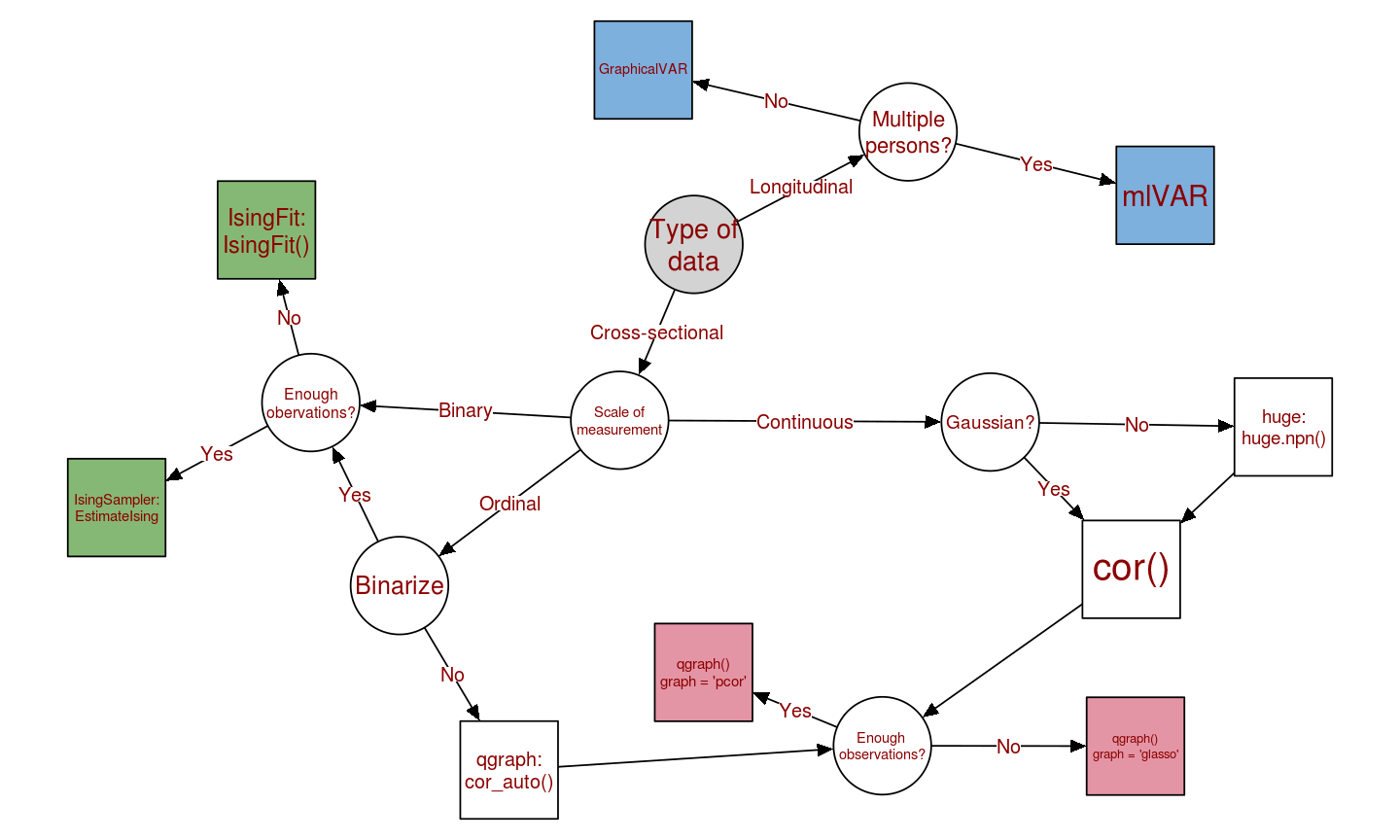## Outline

• Part 1: Markov Random Fields
• Gaussian Graphical Model
• Ising Model
• LASSO regularization
• Part 2: Residual Interaction Modeling
• Combining factor analysis and network modeling
• rim demonstration
• Part 3: Longitudinal analysis
• Single person: GraphicalVAR
• Multiple persons: mlVAR

## Networks

• A network is a set of nodes connected by a set of edges
• Nodes represent variables
• Edges can be directed or undirected and represent interactions
• Color and width indicate the strength and sign of an edge (Epskamp et al. 2012)

## Directed network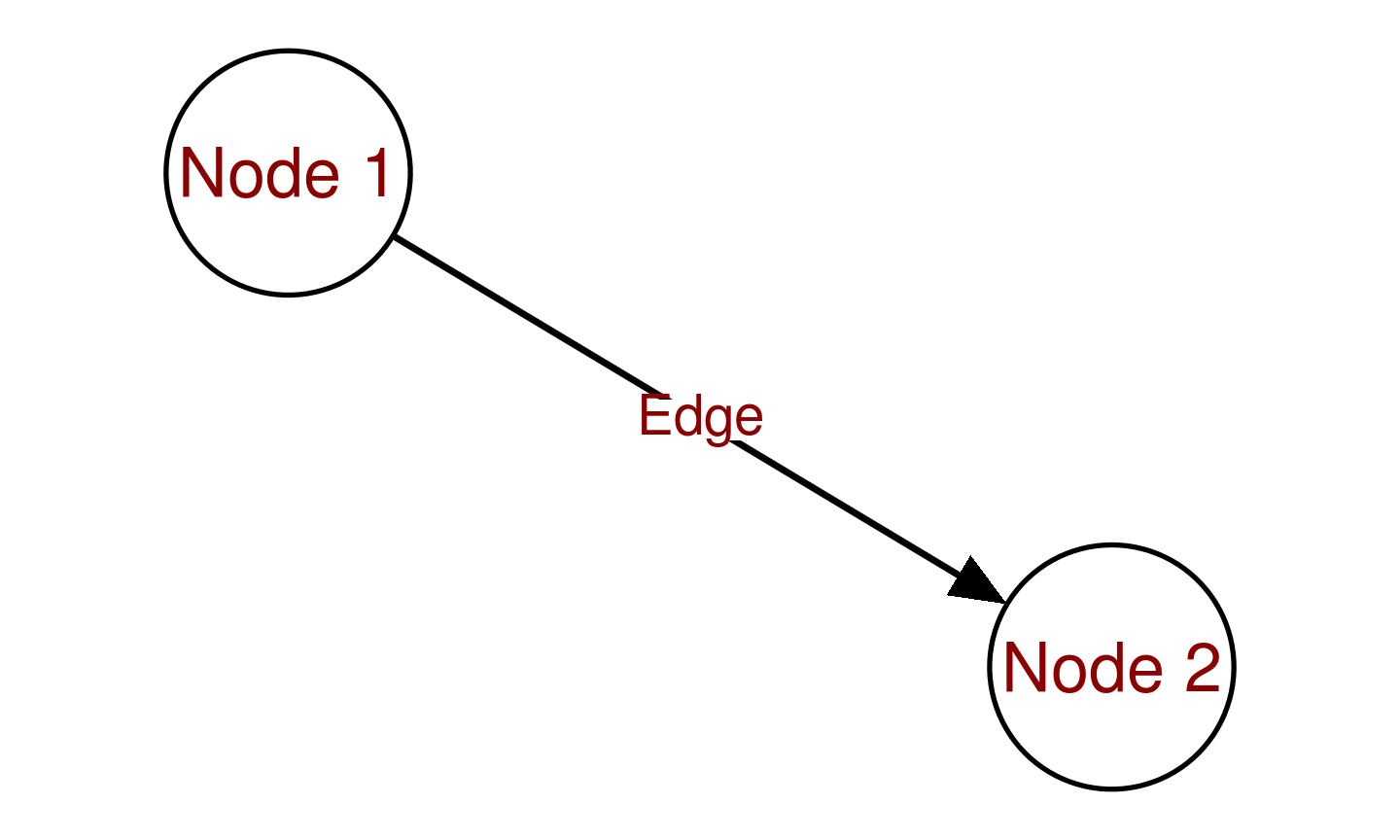## Undirected network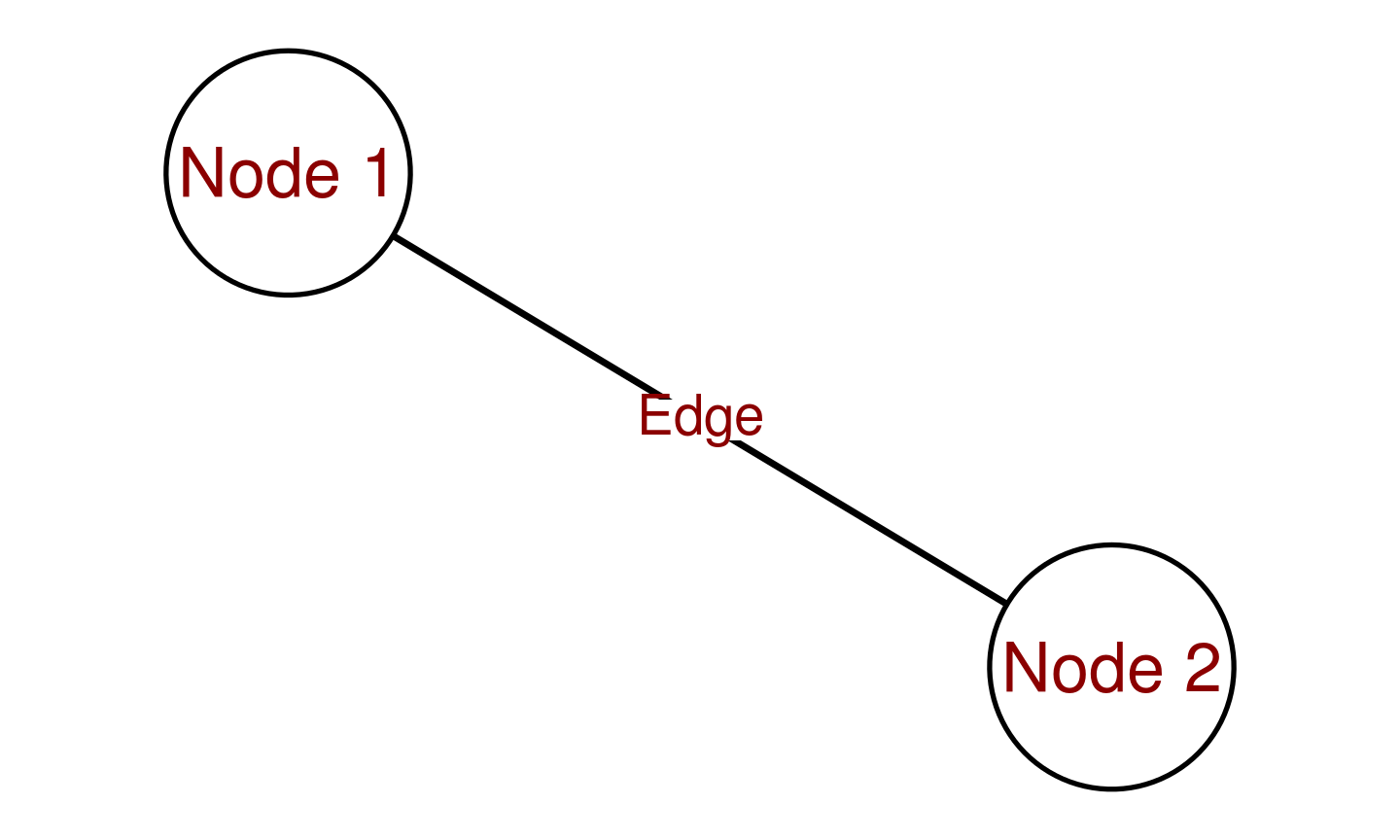## Weighted network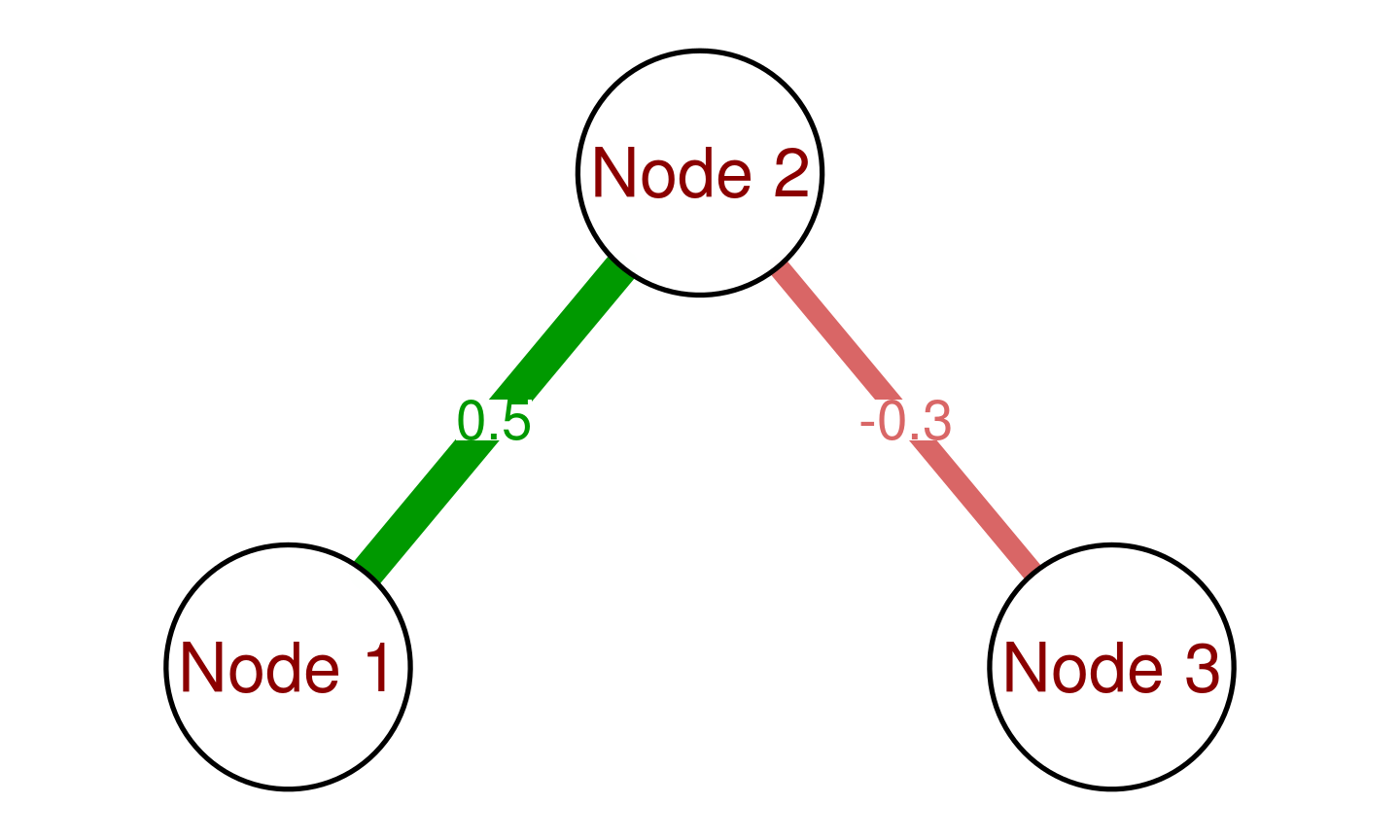## Markov Random Fields

• A pairwise Markov Random Field (MRF) is an undirected network
• Two nodes are connected if they are not independent conditional on all other nodes.
• More importantly, two nodes are NOT connected if they are independent conditioned on all nodes:
• $$X_i \!\perp\!\!\!\perp X_j \mid \boldsymbol{X}^{-(i,j)} = \boldsymbol{x}^{-(i,j)} \iff (i,j) \not\in E$$
• A node separates two nodes if it on all paths from one node to another
• Assumption: only pairwise effects
• No equivalent models!
• Clear saturated model is a fully connected network
• Naturally cyclic!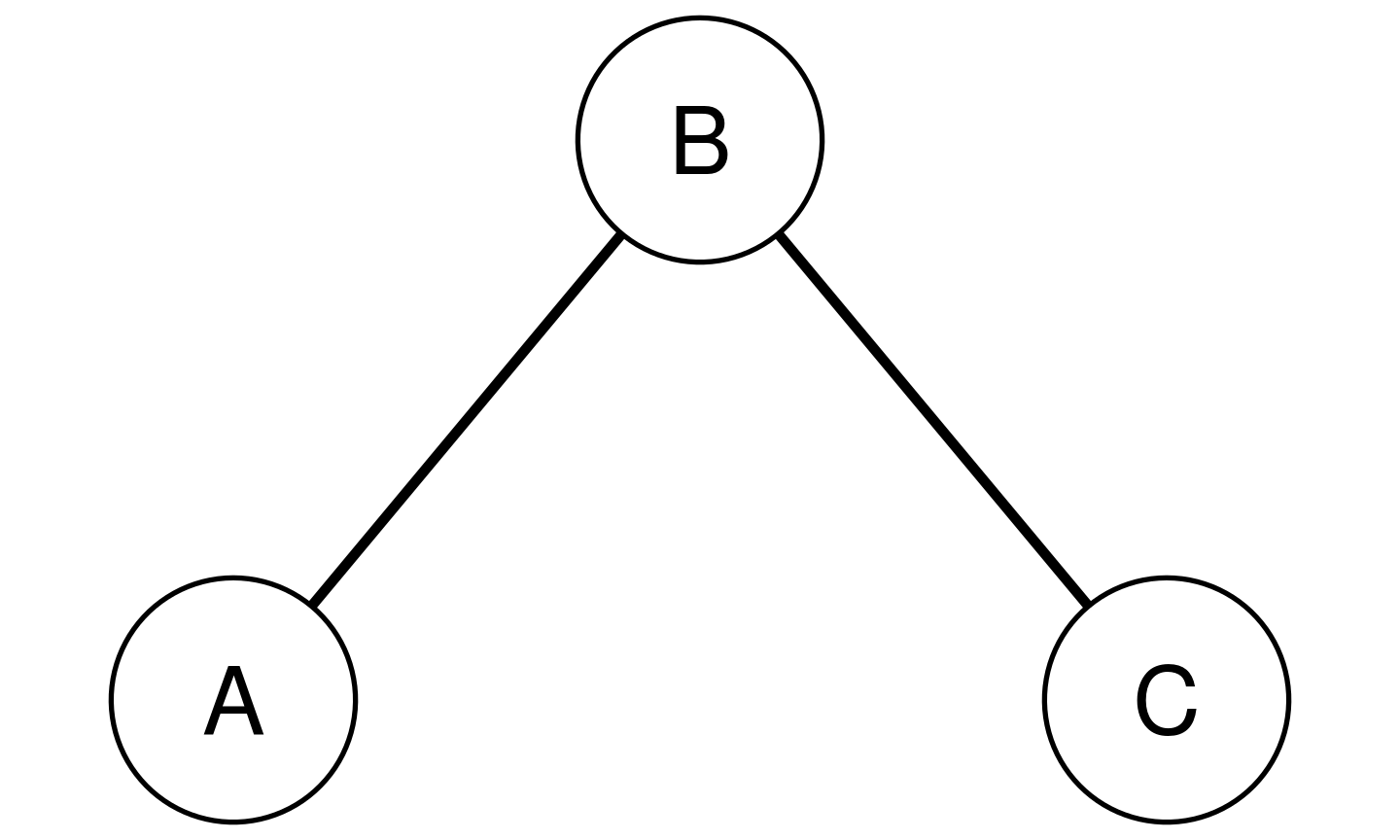• $$B$$ separates $$A$$ and $$C$$
• $$A \!\perp\!\!\!\perp C \mid B$$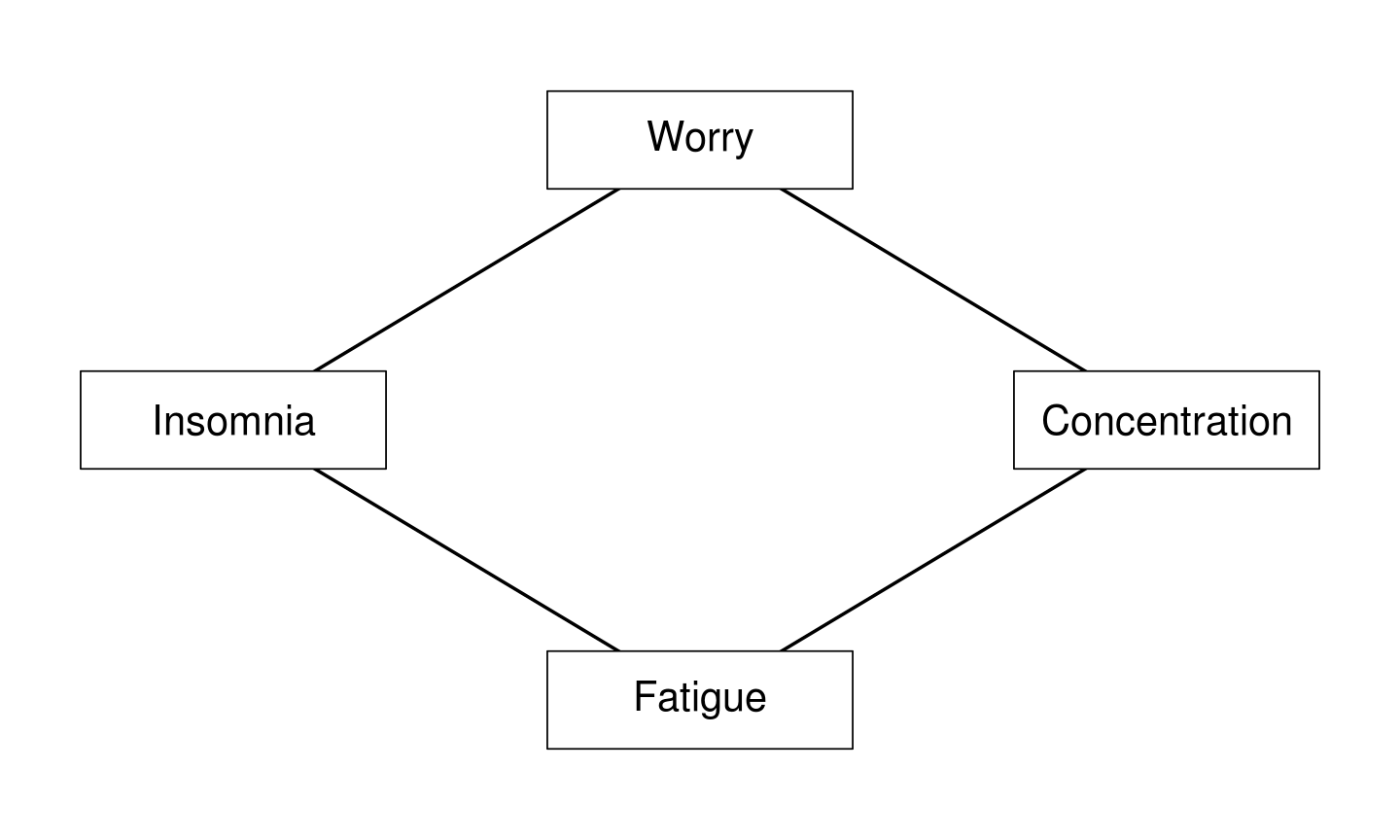• Worrying and fatigue separate Insomnia and Concentration

## Predictive Effects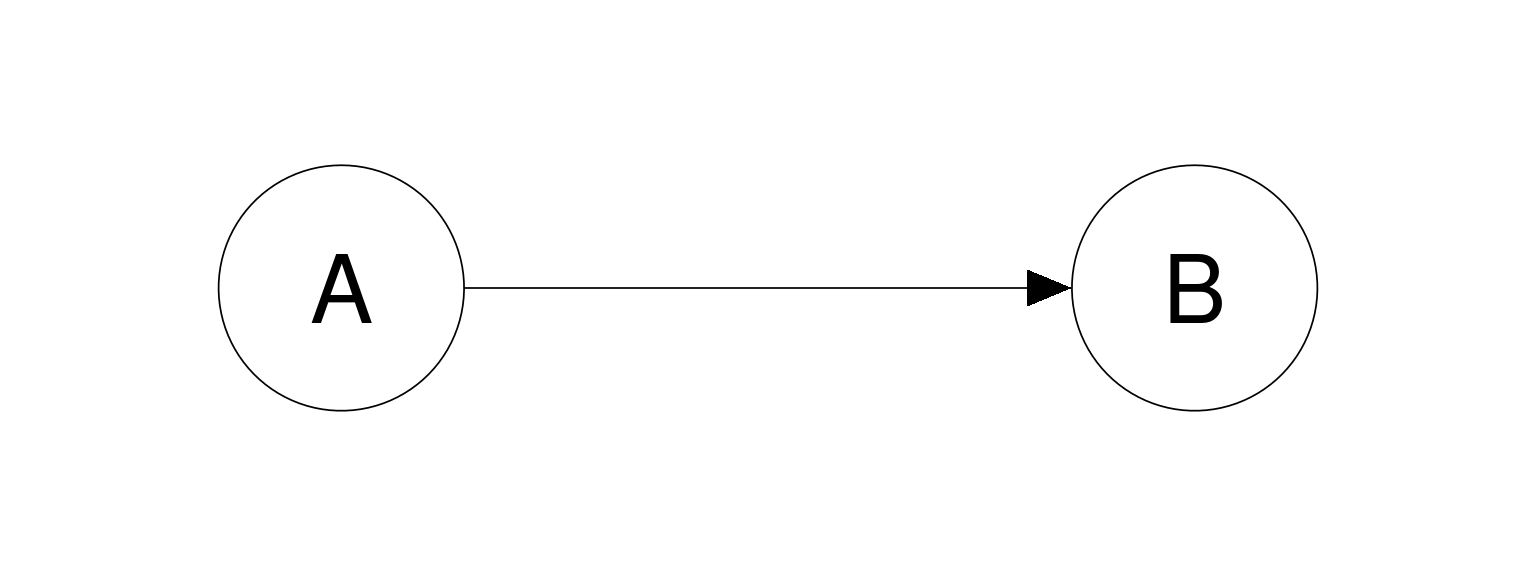If this model is the generating model, does:

• $$A$$ predict $$B$$?
• Yes!
• $$B$$ predict $$A$$?
• Yes!
• $$A$$ predict $$B$$ just as well as $$B$$ predict $$A$$?
• Using linear or logistic regression, yes!
# Generate data (binary):
A <- sample(c(0,1), 10000, replace = TRUE)
B <- 1 * (runif(10000) < ifelse(A==1, 0.8, 0.2))

# Predict A from B (logistic bregression):
AonB <- glm(A ~ B, family = "binomial")
coef(AonB)
## (Intercept)           B
##   -1.369453    2.757481
# Predict B from A (logistic regression):
BonA <- glm(B ~ A, family = "binomial")
coef(BonA)
## (Intercept)           A
##   -1.363489    2.757481
• The logistic regression parameters are equal!

## Predictive Effects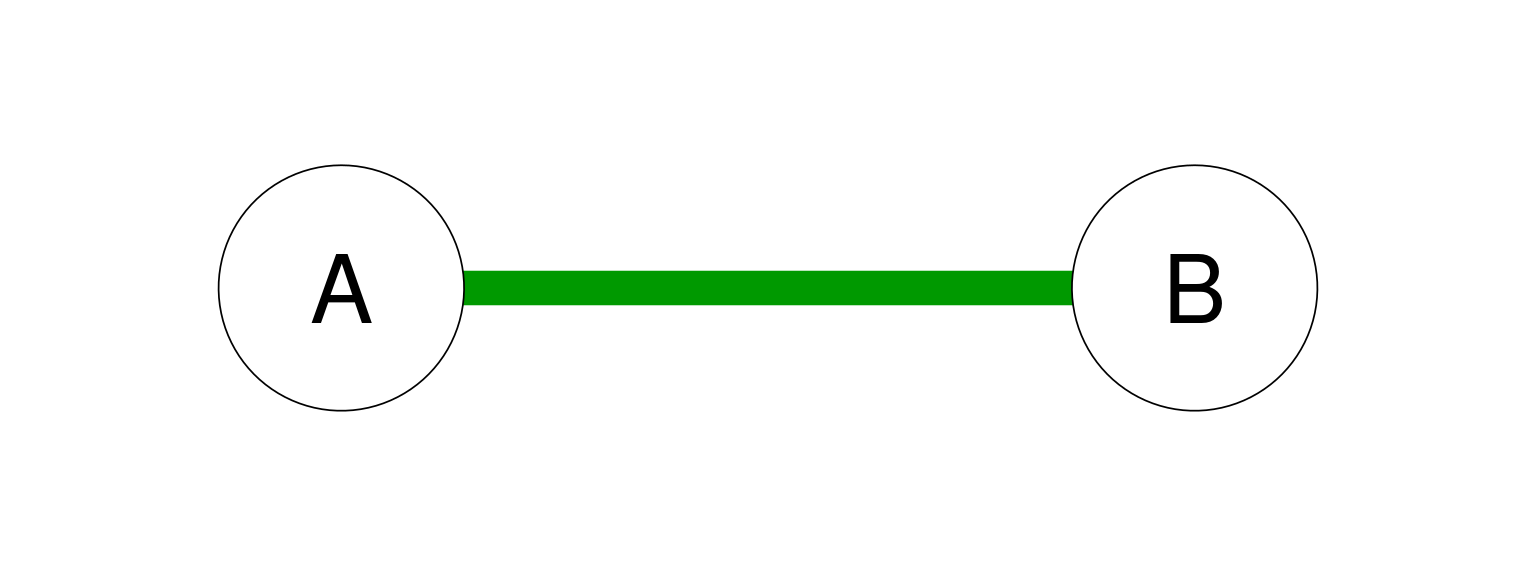• $$A$$ predicts $$B$$ and $$B$$ predicts $$A$$

## Predictive Effects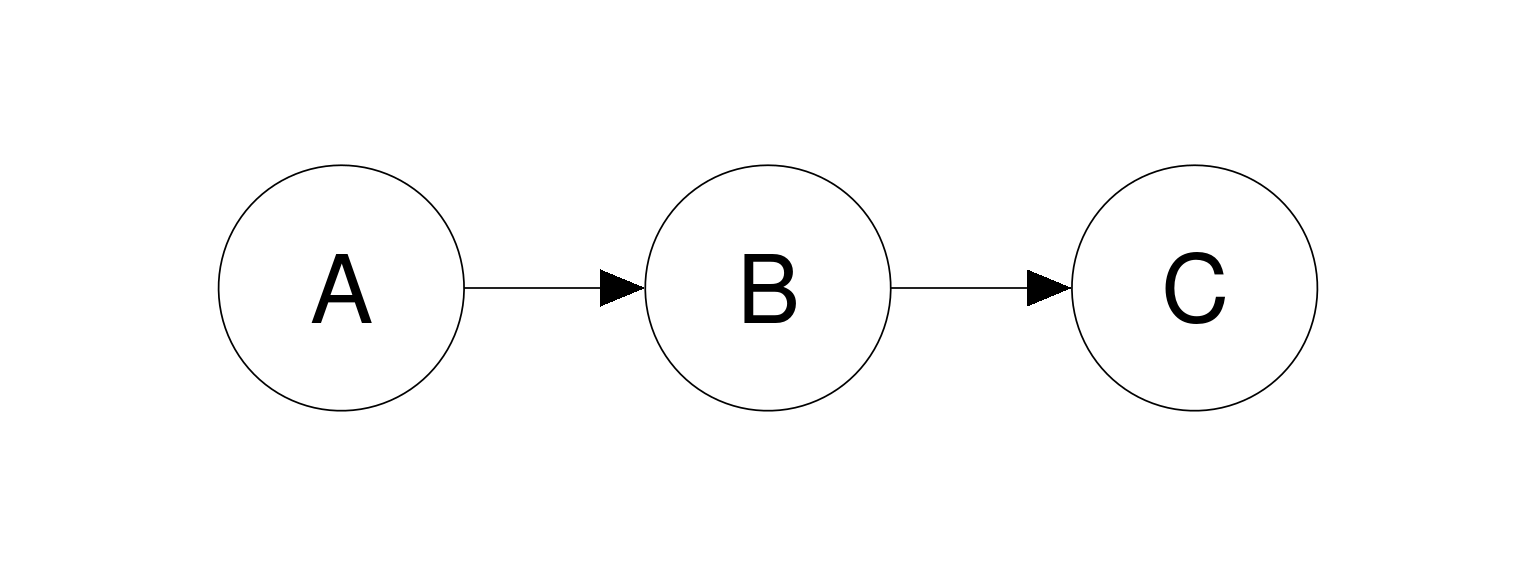If this model is the generating model, does:

• $$A$$ predict $$C$$ or vise versa?
• Yes, they are correlated
• $$A$$ predict $$C$$ or vise versa when also taking $$B$$ into account?
• No!
• In a multiple (logistic) regression, $$C$$ should not predict $$A$$ when $$B$$ is also taken as predictor
# Generate data (Gaussian):
A <- rnorm(10000)
B <- A + rnorm(10000)
C <- B + 2*rnorm(10000)
# Predict A from C:
AonC <- lm(A ~ C)
summary(AonC)
##
## Call:
## lm(formula = A ~ C)
##
## Residuals:
##     Min      1Q  Median      3Q     Max
## -3.2389 -0.6162  0.0059  0.6132  3.3662
##
## Coefficients:
##              Estimate Std. Error t value Pr(>|t|)
## (Intercept) -0.003797   0.009093  -0.418    0.676
## C            0.170163   0.003742  45.469   <2e-16 ***
## ---
## Signif. codes:  0 '***' 0.001 '**' 0.01 '*' 0.05 '.' 0.1 ' ' 1
##
## Residual standard error: 0.9092 on 9998 degrees of freedom
## Multiple R-squared:  0.1713, Adjusted R-squared:  0.1713
## F-statistic:  2067 on 1 and 9998 DF,  p-value: < 2.2e-16
# Predict A from B and C:
AonBC <- lm(A ~ B + C)
summary(AonBC)
##
## Call:
## lm(formula = A ~ B + C)
##
## Residuals:
##      Min       1Q   Median       3Q      Max
## -2.66254 -0.47831 -0.00956  0.47492  2.53815
##
## Coefficients:
##              Estimate Std. Error t value Pr(>|t|)
## (Intercept) -0.005440   0.007056  -0.771    0.441
## B            0.494626   0.006086  81.269   <2e-16 ***
## C            0.003341   0.003556   0.939    0.348
## ---
## Signif. codes:  0 '***' 0.001 '**' 0.01 '*' 0.05 '.' 0.1 ' ' 1
##
## Residual standard error: 0.7056 on 9997 degrees of freedom
## Multiple R-squared:  0.501,  Adjusted R-squared:  0.5009
## F-statistic:  5019 on 2 and 9997 DF,  p-value: < 2.2e-16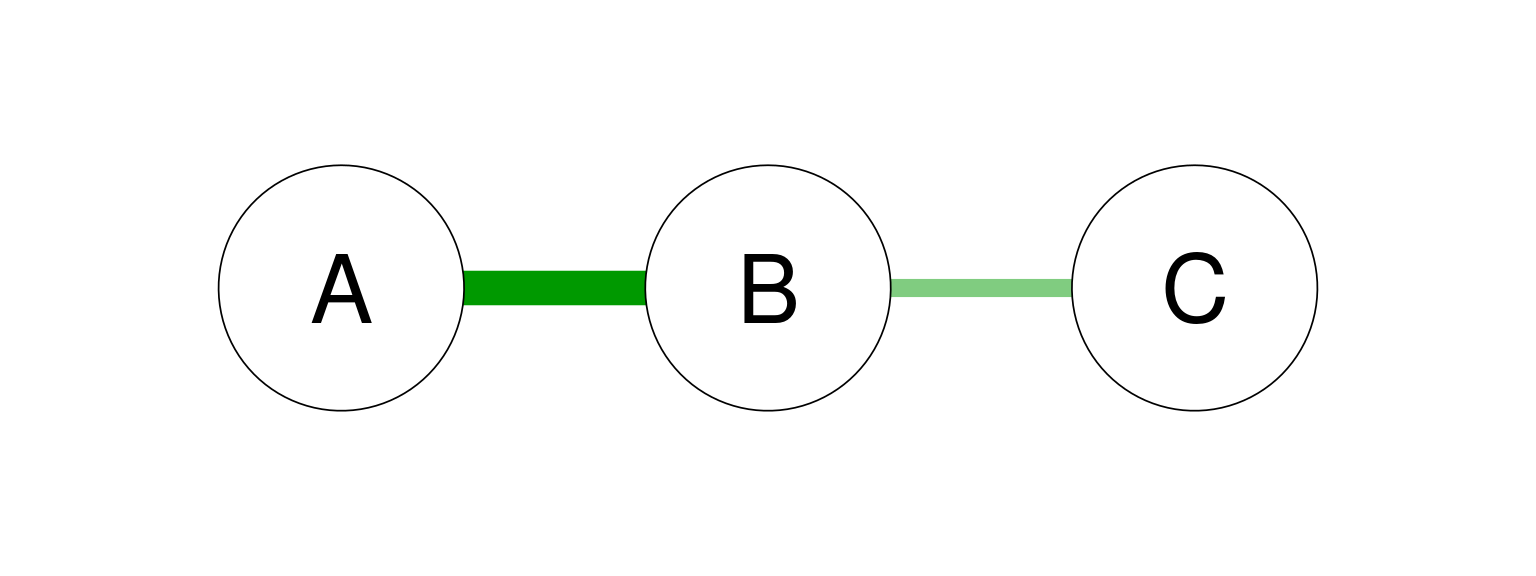• $$A$$ predicts $$B$$ better than $$C$$ predicts $$B$$
• The relationship between $$A$$ and $$C$$ is mediated by $$B$$

## Conditional Independency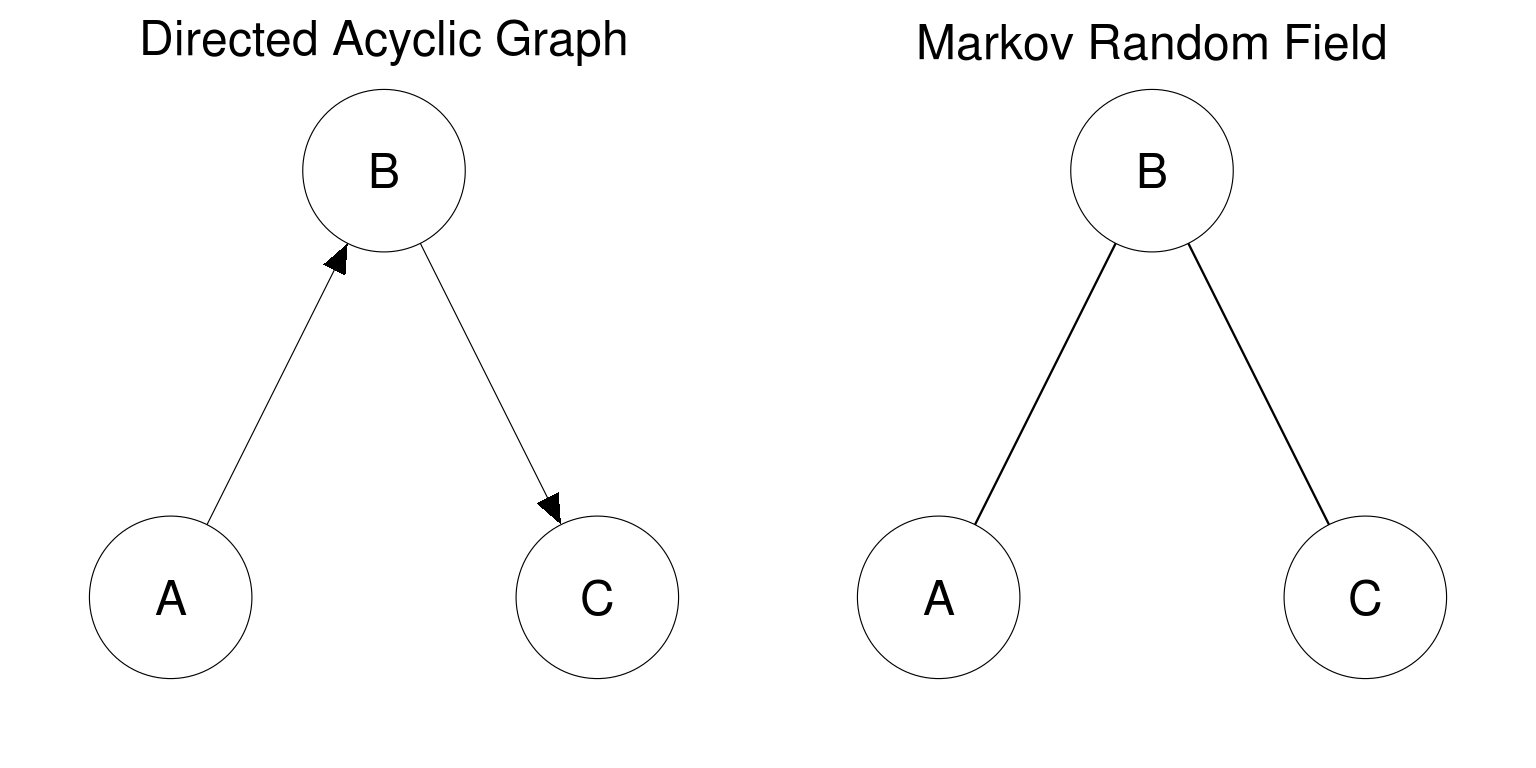## Conditional Independency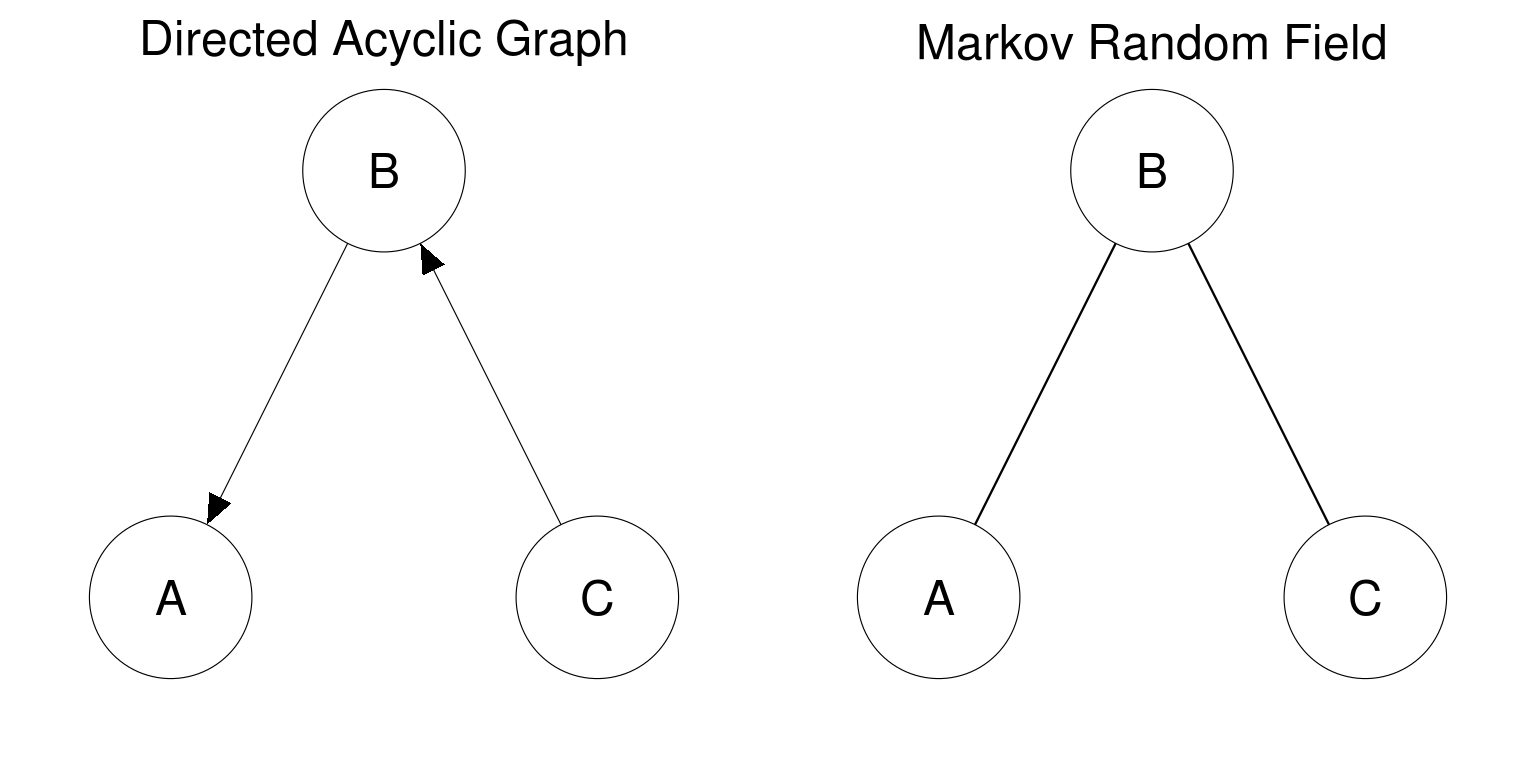## Conditional Independency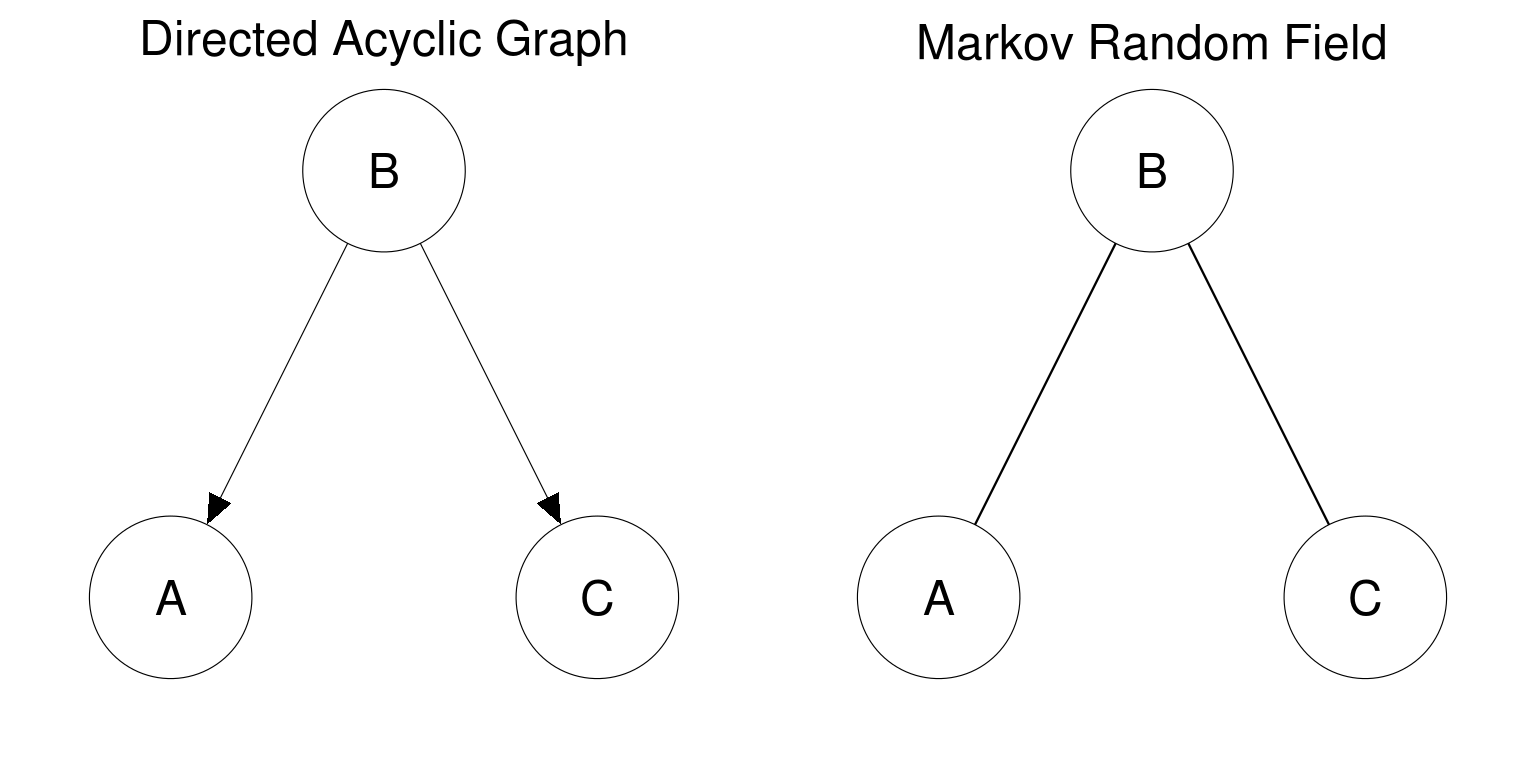## Conditional Independency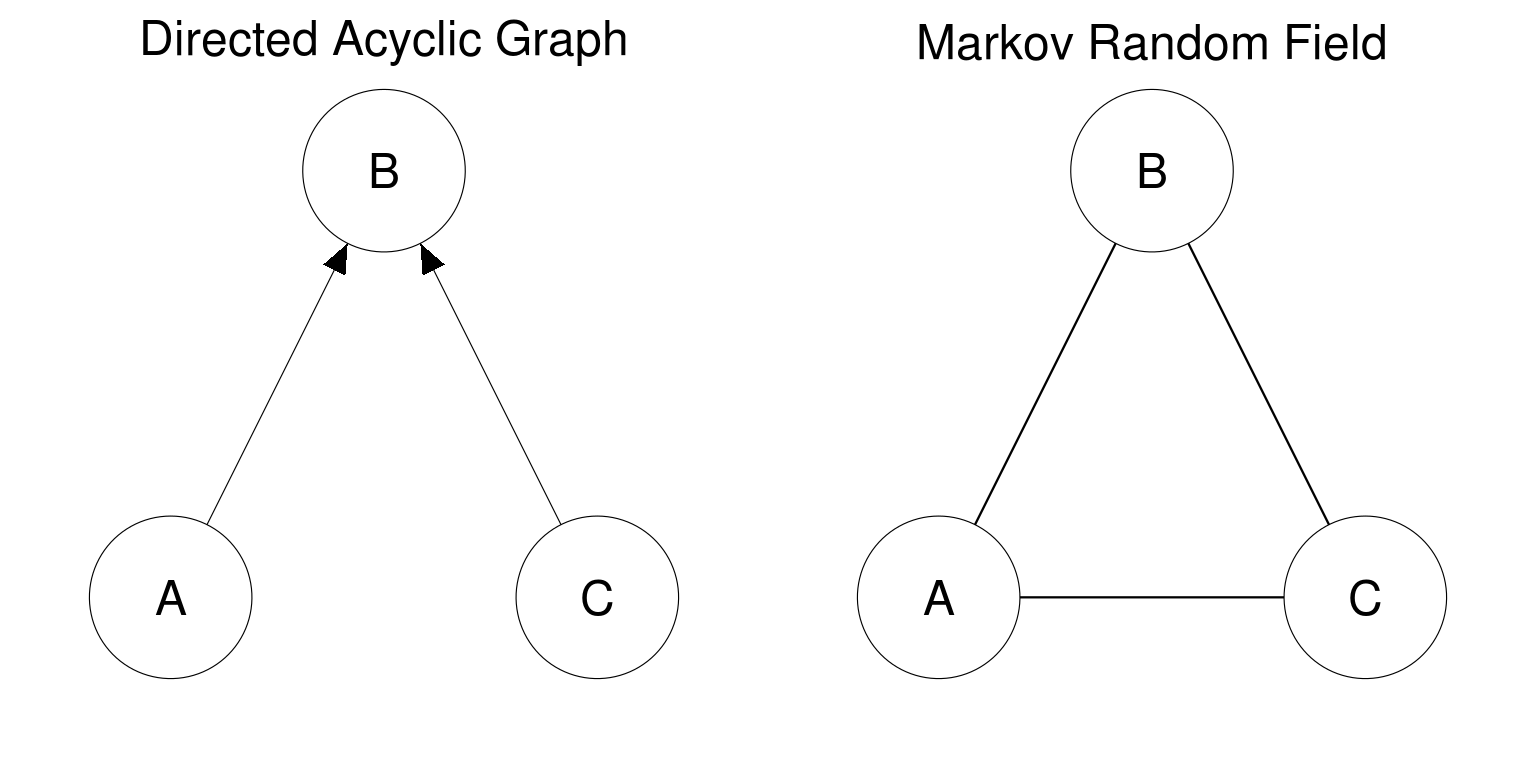• A MRF can not represent the exact implied independence relationship of a collider structure
• Three edges are needed instead of two
• However, exogenous variables are commonly modeled to be correlated anyway